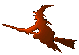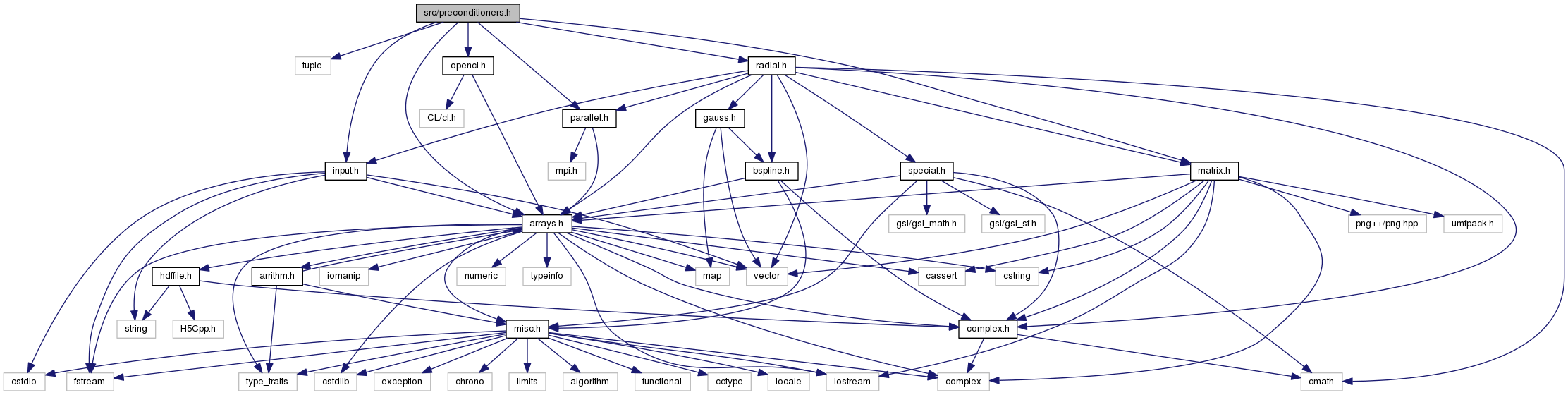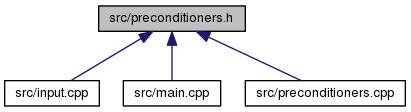Hex  1.0 Hydrogen-electron collision solver
preconditioners.h File Reference
#include <tuple>
#include "arrays.h"
#include "input.h"
#include "matrix.h"
#include "parallel.h"
#include "opencl.h"
Include dependency graph for preconditioners.h:This graph shows which files directly or indirectly include this file:Go to the source code of this file.

Data Structures

class  PreconditionerBase
Preconditioner template. More...

class  NoPreconditioner
Solution driver without actual preconditioner. More...

class  CGPreconditioner
CG iteration-based preconditioner. More...

class  GPUCGPreconditioner
CG iteration-based preconditioner (GPU variant). More...

class  JacobiCGPreconditioner
Jacobi-preconditioned CG-based preconditioner. More...

class  SSORCGPreconditioner
SSOR-preconditioned CG-based preconditioner. More...

class  ILUCGPreconditioner
ILU-preconditioned CG-based preconditioner. More...

class  DICCGPreconditioner
DIC-preconditioned CG-based preconditioner. More...

class  SPAICGPreconditioner
SPAI-preconditioned CG-based preconditioner. More...

class  TwoLevelPreconditioner
Two-resolution preconditioner. More...

class  MultiresPreconditioner
Multi-resolution (V-cycle) preconditioner. More...

class  Preconditioners
Preconditioner traits. More...

Functions

cArray iChol (cArrayView const &A, lArrayView const &I, lArrayView const &P)
Sparse incomplete Cholesky decomposition. More...

SymDiaMatrix DIC (SymDiaMatrix const &A)
DIC preconditioner. More...

SymDiaMatrix SSOR (SymDiaMatrix const &A)
SSOR preconditioner. More...

CooMatrix SPAI (SymDiaMatrix const &A, const iArrayView diagonals)
SPAI preconditioner. More...

Function Documentation

 SymDiaMatrix DIC ( SymDiaMatrix const & A )

Setup the diagonal incomplete Cholesky preconditioner. It is a essentially the original matrix with a preconditioned diagonal and the strict upper and lower triangles normalized by the preconditioned diagonal, i.e. a matrix

$\mathbf{P} = \mathbf{\tilde{L}}_\mathbf{A} + \mathbf{D}^{-1} + \mathbf{\tilde{L}}_\mathbf{A}^T$

for the preconditioner

$\mathbf{M} = (\mathbf{D} + \mathbf{L}_\mathbf{A}) \mathbf{D}^{-1} (\mathbf{D} + \mathbf{U}_\mathbf{A}) = (1 + \mathbf{\tilde{U}}_\mathbf{A}^T) \mathbf{D} (1 + \mathbf{\tilde{U}}_\mathbf{A})$

The formula for the elements of $$\mathbf{D}$$ is

$d_i = a_{ii} - \sum_{k < i} a_{ik} d_{k}^{-1} a_{ki} \ ,$

and is to be evaluated along the diagonal, re-using the just computed values $$d_i$$. Hence, the access pattern in dense matrix would be

$\pmatrix { \ast & & & \ast & & \cr & \ast & & \ast & & \cr & & \ast & \ast & & \cr \ast & \ast & \ast & ? & & \cr & & & & & \cr & & & & & \cr }$

In the case of the sparse SymDiaMatrix, the asterisks will occur only on the nonzero diagonals.

Note
The same preconditioner can be used for unsymmetric matrix. Then it is called DILU (diagonal incomplete LU factorization). This function, though, is implemented symmetrically (as the input is symmetrical by definition of the type).
Parameters
 A Matrix in SymDiaMatrix format that is to be preconditioned.
Returns
The DIC preconditioner of the symmetric matrix.
 cArray iChol ( cArrayView const & A, lArrayView const & I, lArrayView const & P )

This routine computes the LDL-decomposition of a symmetric matrix,

$A = L D L^T \ ,$

where $$L$$ is a lower triangular matrix normalized so that it has units on the diagonal and $$D$$ is a diagonal matrix.

Parameters
 A Matrix elements in the form of a consecutive array $$\left\{a_i\right\}_{i=1}^N$$ as in $\pmatrix { a_1 & & & & \cr a_2 & a_3 & & & \cr a_4 & a_5 & a_6 & & \cr a_7 & a_8 & a_9 & a_{10} & \cr \vdots & & & & \ddots \cr }$ Whenever $$a_k$$ is equal to zero, it is (or can be) omitted from the input array. I Array of column indices (one for every element of A). P Array of row pointers, i.e. starting positions of rows of A. For dense matrix it would be 0, 1, 3, 6, 10, ... The last element must be equal to the length of both A and I.
Returns
The elements of $$L$$ (below diagonal) and $$D$$ (at diagonal) with the exact sparse pattern as the input array A, i.e. specified by I and P arrays.
 CooMatrix SPAI ( SymDiaMatrix const & A, const iArrayView diagonals )

Compute sparse aproximate inverse of a given symmetrix diagonal matrix A. The sparse structure of the SPAI is set by the second parameter that contains list of non-lower diagonal indices (greater than or equal to zero).

This function uses Lapack routine ZGELSD.

 SymDiaMatrix SSOR ( SymDiaMatrix const & A )

Symmetric successive over-relaxation preconditioner for $$\omega = 1$$. (Essentially symmetrized Gauss-Seidel). The resulting matrix contains normalized lower (and upper) triangle and in the place of the unit diagonal is the inverse diagonal of $$\mathbf{A}$$. So, having the preconditioner